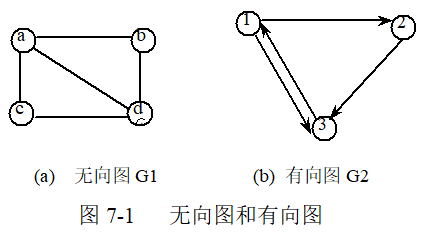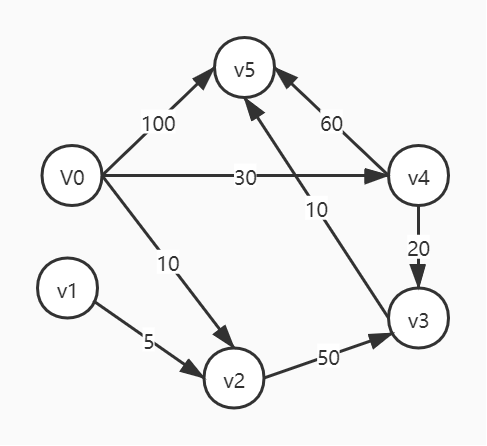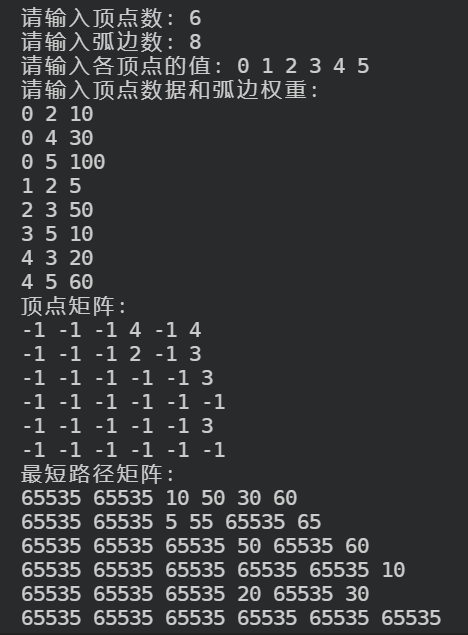## 基本概念

### 图

• 图是由顶点集 V 和顶点间的关系集合 E （边的集合）组成的一种数据结构。
• 用二元组定义为：G = (V, E)G1 和 G2 的数据结构分别可以表示为：

• G1 = (V1, E1)，其中 V1 = {a, b, c, d}，E1 = {(a, b), (a, c), (a, d), (b, d), (c, d)}
• G2 = (V2, E2)，其中 V2 = {1, 2, 3}，E2 = {<1, 2>, <1, 3>, <2, 3>, <3, 1>}

## 算法分析

dis<vi, vj> 为顶点 vivj 的距离，若能找到一个中介顶点 vk，使得 dis<vi, vk> + dis<vk, vj> < dis<vi, vj>，则更新 dis<vi, vj> 的值为 dis<vi, vk> + dis<vk, vj>• 规定顶点到自身的距离为无穷大。
• 邻接矩阵表示相邻顶点之间的关系，也就是说，如果两个顶点不能直接到达，则这两个顶点之间的距离为无穷大。
v0 v1 v2 v3 v4 v5
v0 \infty \infty 10 \infty 30 100
v1 \infty \infty 5 \infty \infty \infty
v2 \infty \infty \infty 50 \infty \infty
v3 \infty \infty \infty \infty \infty 10
v4 \infty \infty \infty 20 \infty 60
v5 \infty \infty \infty \infty \infty \infty

v0 v1 v2 v3 v4 v5
v0 \infty \infty 10 60 30 100
v1 \infty \infty 5 55 \infty \infty
v2 \infty \infty \infty 50 \infty \infty
v3 \infty \infty \infty \infty \infty 10
v4 \infty \infty \infty 20 \infty 60
v5 \infty \infty \infty \infty \infty \infty

v0 v1 v2 v3 v4 v5
v0 \infty \infty 10 60 30 70
v1 \infty \infty 5 55 \infty 65
v2 \infty \infty \infty 50 \infty 60
v3 \infty \infty \infty \infty \infty 10
v4 \infty \infty \infty 20 \infty 30
v5 \infty \infty \infty \infty \infty \infty

v0 v1 v2 v3 v4 v5
v0 \infty \infty 10 50 30 60
v1 \infty \infty 5 55 \infty 65
v2 \infty \infty \infty 50 \infty 60
v3 \infty \infty \infty \infty \infty 10
v4 \infty \infty \infty 20 \infty 30
v5 \infty \infty \infty \infty \infty \infty

## 算法流程

• 构建有向网
• 输入顶点个数和弧边数；
• 输入各顶点的值；
• 初始化邻接矩阵的值，默认为无穷大；
• 输入各个弧边的起始顶点，结束顶点以及边的权值。
• 弗洛伊德算法
• 初始化数组 P 和数组 D，数组 P 用来存储最短路径中经过的顶点的下标，数组 D 用来保存各个顶点之间的最短路径；
• 遍历每一个顶点 K，将顶点 K 作为中介结点，计算从开始顶点 A 到到顶点 K，再从顶点 K 到结束顶点 B 的所有弧边权值之和是否小于顶点 A 到顶点 B 之间的距离，若小于，则修改顶点 A 到顶点 B 之间的距离为更小值。

## 代码实现

#include <iostream>
using namespace std;

#define MAX_VERTEX_NUM 30 // 最大顶点数
#define INFINITY 65535    // 表示无穷大

typedef int VRType;                                         // 弧的权值类型
typedef int VertexType;                                     // 图中顶点的数据类型
typedef int PathMatrix[MAX_VERTEX_NUM][MAX_VERTEX_NUM];     // 用于存储最短路径中经过的顶点下标
typedef int ShortPathTable[MAX_VERTEX_NUM][MAX_VERTEX_NUM]; // 用于存储各个顶点之间的最短路径

class MGraph
{
private:
VertexType vexs[MAX_VERTEX_NUM];             // 存储中顶点数据
VRType arcs[MAX_VERTEX_NUM][MAX_VERTEX_NUM]; // 二维数组，记录顶点之间的关系（弧边的权值）
int vexNum;                                  // 顶点数
int arcNum;                                  // 弧边数

public:
MGraph() {}
int getVexNum() // 获取顶点数
{
return vexNum;
}
int getArcNum() // 获取弧边数
{
return arcNum;
}
int locateVex(MGraph G, VertexType v);                              // 根据顶点数据，找到顶点所在位置
void createUDG(MGraph &G);                                          // 构造网
void shortestPathFloyd(MGraph G, PathMatrix &P, ShortPathTable &D); // 弗洛伊德算法
};

// 根据顶点数据，找到顶点所在位置
int MGraph::locateVex(MGraph G, VertexType v)
{
int i = 0;
while (i < G.vexNum)
{
if (G.vexs[i] == v) // 找到位置，直接返回下标
{
return i;
}
i++;
}
return -1; // 未找到，返回 - 1
}

// 构造网
void MGraph::createUDG(MGraph &G)
{
cout << "请输入顶点数：";
cin >> G.vexNum;
cout << "请输入弧边数：";
cin >> G.arcNum;

cout << "请输入各顶点的值：";
for (int i = 0; i < G.vexNum; i++)
{
cin >> G.vexs[i];
}

// 初始化 G.arcs 数组，默认值为 INFINITY
for (int i = 0; i < G.vexNum; i++)
{
for (int j = 0; j < G.vexNum; j++)
{
G.arcs[i][j] = INFINITY;
}
}

cout << "请输入顶点数据和弧边权重：" << endl;
for (int i = 0; i < G.arcNum; i++)
{
int v1, v2, w;
cin >> v1 >> v2 >> w;
int n = locateVex(G, v1);
int m = locateVex(G, v2);

if (n == -1 || m == -1)
{
cout << "不存在该顶点！" << endl;
return;
}
G.arcs[n][m] = w;
}
}

// 弗洛伊德算法
void MGraph::shortestPathFloyd(MGraph G, PathMatrix &P, ShortPathTable &D)
{
// 初始化数组 P 和 D
for (int v = 0; v < G.vexNum; v++)
{
for (int w = 0; w < G.vexNum; w++)
{
D[v][w] = G.arcs[v][w]; // D 数组元素初始值为邻接矩阵的值
P[v][w] = -1;           // P 数组元素默认值为 -1
}
}

// 拿出每个顶点作为遍历条件
for (int k = 0; k < G.vexNum; k++)
{
for (int v = 0; v < G.vexNum; v++)
{
for (int w = 0; w < G.vexNum; w++)
{
// 判断经过顶点 k 的距离是否更短，若更短，则存储更短的路径
if (D[v][k] + D[k][w] < D[v][w])
{
D[v][w] = D[v][k] + D[k][w];
P[v][w] = k;
}
}
}
}
}

int main()
{
MGraph G;
G.createUDG(G); // 创建有向网

PathMatrix P;
ShortPathTable D;
G.shortestPathFloyd(G, P, D); // 弗洛伊德算法

// 打印顶点矩阵
cout << "顶点矩阵：" << endl;
for (int i = 0; i < G.getVexNum(); i++)
{
for (int j = 0; j < G.getVexNum(); j++)
{
cout << P[i][j] << " ";
}
cout << endl;
}

// 打印最短路径矩阵
cout << "最短路径矩阵：" << endl;
for (int i = 0; i < G.getVexNum(); i++)
{
for (int j = 0; j < G.getVexNum(); j++)
{
cout << D[i][j] << " ";
}
cout << endl;
}

return 0;
}